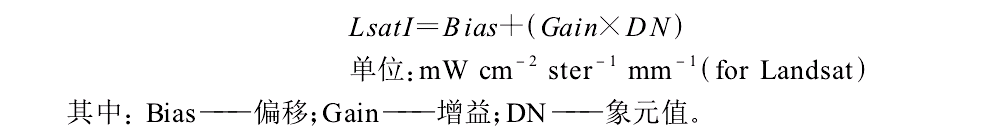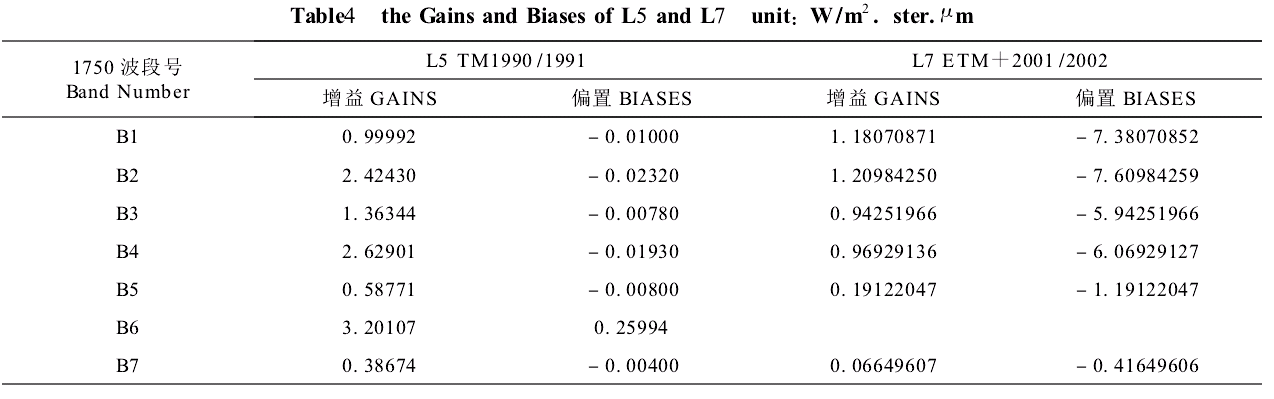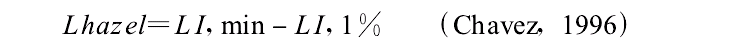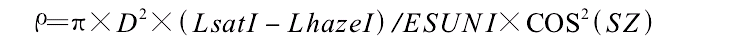# Python 处理遥感图像：光谱辐射定标、大气校正和计算反射率

①、遥感影像的光谱辐射定标def computL(gain,Dn,bias):
return (gain*Dn+bias)

②、遥感影像的大气校正#ESUN
ESUNI71=196.9
#
Lmini=-6.2
Lmax=293.7
#
Qcal=1
Qmax=255
LIMIN=Lmini+(Qcal*(Lmax-Lmini)/Qmax)
LI=(0.01*ESUNI71*cos*cos)/(math.pi*D*D)
Lhazel=LIMIN-LI



③、计算遥感影像的反射率（JD为遥感成像的儒略日(Julian Day)，计算公式为：
JD=K-32075+1461*(I+4800+(J-14)/12)/4+367*(J-2-(J-14)/12*12)/12-3*((I+4900+(J-14)/12)/100)/4

I、J、K分别为年、月、日

import cv2
import numpy as np
import math

#图像格式转换
img10=cv2.cvtColor(img1,cv2.COLOR_BGR2GRAY)

#计算JD
I=2003
J=2
K=20
JD=K-32075+1461*(I+4800+ (J-14)/12)/4+367*(J-2-(J-14)/12*12)/12-3*((I+4900+(J-14)/12)/100)/4
#设置ESUNI值
ESUNI71=196.9
#计算日地距离D
D=1-0.01674*math.cos((0.9856*(JD-4)*math.pi/180))
#计算太阳天顶角

inter=(math.pi*D*D)/(ESUNI71*cos*cos)

#大气校正参数设置
Lmini=-6.2
Lmax=293.7
Qcal=1
Qmax=255
LIMIN=Lmini+(Qcal*(Lmax-Lmini)/Qmax)
LI=(0.01*ESUNI71*cos*cos)/(math.pi*D*D)
Lhazel=LIMIN-LI

def copy(img,new1):
new1= np.zeros(img.shape,dtype='uint16')
new1[:,:] = img[:,:]

def computL(gain,Dn,bias):
return (gain*Dn+bias)

if __name__ == '__main__':
print 'D=',D
print 'cosZS=',cos
print  'Lhazel=',Lhazel
#计算图像反射率
result=np.zeros(img.shape,dtype='uint16')
for i in range(0,img.shape(1)):
for j in range(0,img.shape(0)):
Lsat=computL(1.18070871,img10[i,j],-7.38070852)
result[i,j]=inter*(Lsat-Lhazel)*1000

#保存图像
cv2.imwrite("F:\\result.tif", result)
cv2.namedWindow("Image")
cv2.imshow("Image", result)
cv2.waitKey(0)


12-271731
06-2024610-301万+
11-122万+
06-306402
10-241万+
08-31
12-06
12-03